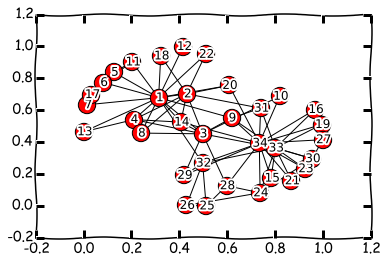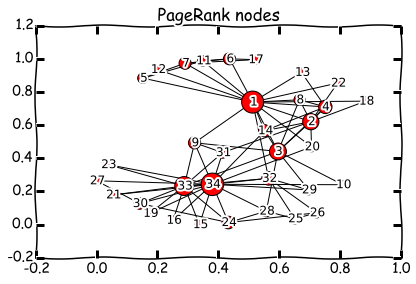# Lecture 8: Eigenvalues (and eigenvectors)¶

## Syllabus¶

Week 1: Matrices, vectors, matrix/vector norms, scalar products & unitary matrices Week 2: TAs-week (Strassen, FFT, a bit of SVD) Week 3: Matrix ranks, singular value decomposition, linear systems, eigenvalues

## Recap of the previous lecture¶

• Linear systems
• Gaussian elimination
• Sparse matrices
• and the condition number!

## Today lecture¶

• Eigenvectors and their applications (PageRank)
• Gershgorin circles
• Computing eigenvectors using power method
• Schur theorem
• Normal matrices

## What is an eigenvector¶

An vector $x \ne 0$ is called an eigenvector of a square matrix $A$ if there exists a number $\lambda$ such that
$$Ax = \lambda x.$$ The number $\lambda$ is called an eigenvalue.
The names eigenpair and eigenproblem are also used.

## Eigendecomposition¶

If matrix $A$ of size $n\times n$ has $n$ eigenvectors $s_i$, $i=1,\dots,n$: $$As_i = \lambda_i s_i,$$ then this can be written as $$A S = S \Lambda, \quad\text{where}\quad S=(s_1,\dots,s_n), \quad \Lambda = \text{diag}(\lambda_1, \dots, \lambda_n),$$ or equivalently $$A = S\Lambda S^{-1}.$$ This is called eigendecomposition of a matrix. Matrices that can be represented by their eigendecomposition are called diagonalizable.

#### Existence¶

What classes of matrices are diagonalizable?

Simple example can be matrices with all different eigenvalues. More generally, matrix is diagonalizable iff algebraic multiplicity of each eigenvalue (mutiplicity of eigenvalue in the characteristic polynomial) is equal to its geometric multiplicity (dimension of eigensubspace).

For our purposes the most import class of diagonalizable matrices will be normal matrices: $AA^* = A^* A$. You will learn how to prove that normal matrices are diagonalizable after a few slides (Schur decomposition topic).

#### Examples¶

• You can simpy check that, e.g. matrix $$A = \begin{pmatrix} 1 & 1 \\ 0 & 1 \end{pmatrix}$$ has one eigenvalue $1$ of multimplicity $2$ (since its characteristic polynomial is $p(\lambda)=(1-\lambda)^2$), but only one eigenvector $\begin{pmatrix} c \\ 0 \end{pmatrix}$ and hence is not diagonalizable.

• As you remember for circulant matrices we had convolution theorem $C = F^{-1} \Lambda F$ which is nothing, but eigendecomposition with $S = F^{-1} \equiv \frac{1}{n}F^*$ matrix of eigenvectors.

## Why eigenvectors and eigenvalues are important¶

Eigenvectors are both important auxiliary tools and also play important role in applications.
To start with all our microworld is governed by the Schrodinger equation which is an eigenvalue problem.
$$H \psi = E \psi,$$ where $E$ is the ground state energy, $\psi$ is called wavefunction and $H$ is the Hamiltonian.
More than 50% of the computer power is spent on solving this type of problems for computational material / drug design.

## Eigenvalues are vibrational frequencies¶

A typical computation of eigenvectors / eigenvectors is for studying

• Vibrational computations of mechanical structures
• Model order reduction of complex systems
In :
from IPython.display import YouTubeVideo

Out:

One of the most famous eigenvectors computation is the Google PageRank. It is not actively used by Google nowdays, but it was of the main features in its early stages. The question is how do we rank webpages, which one is important, and which one is not. All we know about the web is which page referrs to which. PageRank is defined by a recursive definition. Denote by $p_i$ the importance of the $i$-th page. Then we define this importance as an average value of all importances of all pages that refer to the current page. It gives us a linear system
$$p_i = \sum_{j \in N(i)} \frac{p_j}{L(j)},$$ where $L(j)$ is the number of outgoing links on the $j$-th page, $N(i)$ are all the neighbours. It can be rewritten as
$$p = G p,$$ or as an eigenvalue problem

$$Gp = 1 p,$$

i.e. the eigenvalue $1$ is already known.

## Demo¶

We can compute it using some Python packages. We will use networkx package for working with graphs that can be installed using
conda install networkx

We will use a simple example of Zachary karate club network. This data was collected in 1977, and is a classical social network dataset.

In :
import numpy as np
%matplotlib inline
import matplotlib.pyplot as plt
plt.xkcd()
import networkx as nx
nx.draw_networkx(kn) #Draw the graphNow we can actually compute the PageRank using the NetworkX built-in function. We also plot the size of the nodes larger if its PageRank is larger.

In :
pr = nx.algorithms.link_analysis.pagerank(kn)
pr_vector = [pr[i+1] for i in xrange(len(pr))]
pr_vector = np.array(pr_vector) * 5000
nx.draw_networkx(kn, node_size=pr_vector, labels=None)
plt.tight_layout()
plt.title('PageRank nodes')

Out:
<matplotlib.text.Text at 0x10f6611d0>## Computation of the eigenvalues via characteristic equations¶

The eigenvalue problem has the form
$$Ax = \lambda x,$$ or $$(A - \lambda I) x = 0,$$ therefore matrix $A - \lambda I$ has non-trivial nullspace and should be singular. That means, that the determinant

$$p(\lambda) = \det(A - \lambda I) = 0.$$

The equation is is called characteristic equations and is a polynomial of order $n$.
The $n$-degree polynomial has $n$ complex roots!

## Remember the determinant¶

The determinant of a square matrix $A$ is defined as

$$\det A = \sum_{\sigma \in S_n} \mathrm{sgn}({\sigma})\prod^n_{i=1} a_{i, \sigma_i},$$

where $S_n$ is the set of all permutations of the numbers $1, \ldots, n$,

and $\mathrm{sgn}$ is the signature of the permutation ( $(-1)^p$ where $p$ is the number of transpositions to be made).

(Checkout video "Executing the determinant" on the Gilbert Strang lecture at MIT)

## Properties of the determinant¶

The determinant has many nice properties:

1. $\det(AB) = \det(A) \det(B)$
2. If we have one row as a sum of two vectors, the determinant is a sum of two determinant
3. "Minor expansion": we can expand the determinant through a selected row.

## How we compute the Schur decomposition¶

Everything is fine, but how you compute the Schur form?

## Summary of todays lecture¶

• Eigenvalues, eigenvectors
• Gershgorin theorem
• Power method
• Schur theorem
• Normal matrices

## Next week¶

Next week we will talk about medium-sized problems and the algorithms hidden in numpy:

SVD, QR, LU, Schur decomposition. How they are actually computed.

Then we will go to sparse and structured matrices.

##### Questions?¶
In :
from IPython.core.display import HTML
def css_styling():